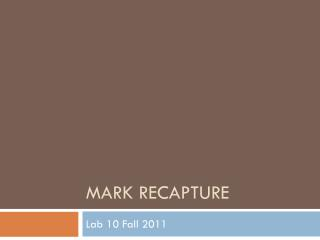Download PresentationMark Recapture

# Mark Recapture - PowerPoint PPT PresentationDownload Presentation## Mark Recapture

- - - - - - - - - - - - - - - - - - - - - - - - - - - E N D - - - - - - - - - - - - - - - - - - - - - - - - - - -
##### Presentation Transcript

1. Mark Recapture Lab 10 Fall 2011

2. Why? • We have 4 goals as managers of wildlife • Increase a population • Decrease a population • Maintain a population • Monitor a population

3. Achieving our 4 goals • What tools do we have thus far to do this? • Map species distributions • Where are they? Where could they be put? • Population growth • How fast are they reproducing? • Population viability • How long can they last? • How many are there? • Distance sampling • Mark - recapture Dave the wildlife manager

4. How many are there? • Mark and recapture is a method commonly used to estimate population size. • fails to detect all individuals present within a population of interest every time • Abundance and density estimation

5. Open vs. Closed • Closed population–no recruitment (birth or immigration) or losses (death or emigration) • Geographic • Demographic • Open population – a population that changes in size and composition from births, deaths, and movements.

6. Capture-recapture models • Closed-population models • two samples – Lincoln-Petersen model  • several samples (k>2) – Schnabel (Schumacher-Eschmeyer) model and models in program CAPTURE. • Open-population models (note: geographic closure is still a critical assumption) • Cormack-Jolly-Seber models (based on k>2) in Program MARK. • Combination of open and closed models (Pollock's Robust Design) in Program MARK.

7. Lincoln-Petersen • A sample of C1 animals is Caught, marked, and released (M2). Later a sample of C2 animals is Captured, of which R2 animals are Recaptures that were previously Marked. • If capture probability (p) is independent of marking status, then the proportion of marked animals in the second sample should be equivalent to the proportion of marked animals in the total population (N) so that R/C = M/N

8. Assumptions • C • L • E • A • R • Rrrr • Population is Closed • Marks are not Lostbetween sample • Animals are Equallylikely to be captured • Marking does not Affectcatchability • Each sample is Random • Recordedcorrectly and Reportedon Recovery

9. Sources of variation • capture probability ( p) = probability of an animal being caught in any trap. • Variation in p comes from?? • heterogeneity • behavior • time

10. Schnabel estimator • Extending the Lincoln-Peterson method • Treating multiple samples as a series of Lincoln-Peterson (L-P) samples and obtained a population estimate as a weighted average of the L-P estimates

11. Assumptions • Same as L-P but assumptions apply to all sampling periods. • same capture probability for a given sampling occasion but capture probabilities can vary amongsampling periods. • BONUS!! Test for violations of assumptions

12. Program CAPTURE • 2CAPTURE, which provides a user-interface to CAPTURE • Need to create the input before you can analyze it • CAPTURE performs abundance and density estimation from information provided by user

13. Notation in output from CAPTURE • t - number of trapping occasions. • n(j) - number of animals captured in the jth sample, j=1,...,t. This is the total number of captures in the experiment. • u(j) - number of new (unmarked) animals captured in the jth sample, j=1,...,t. • f(j) - the capture frequencies. The number of individuals captured exactly j times in t days of trapping j=1,...,t. • M(j) - the number of marked animals in the population at time of the jth sample, j=2,...,t. Note that M(1) equals zero. • M(t+1) - the number of distinct individuals caught during the experiment. • N^ - the estimated population. • p^ - estimated probability of capture. • p^(j) - the estimated probability of capture by occasion. • p-bar - average probability of capture for the generalized removal model. • c^ - estimated probability of recapture.